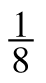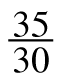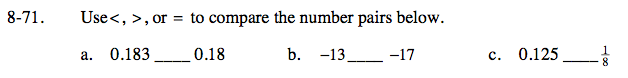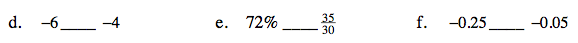Home > ACC7 > Chapter cc38 > Lesson cc38.2.2 > Problem8-71

8-71.
1. Use <, >, or = to compare the number pairs below. Homework Help ✎

1. 0.183 ____ 0.18

2. −13____ −17

3. 0.125 ____4. −6____ −4

5. 72%____6. −0.25____ −0.05Notice that 0.18 can also be looked at as 0.180. This may make the numbers easier to compare.

>

Although 17 is a larger number, since it is negative, it is farther left on the number line than −13. This means that −13 is larger than −17.

>

Converting the fraction into a decimal will make this relationship easier to compare.See (b).

Converting the fraction into a percent will make this relationship easier to compare.

<

See (b).